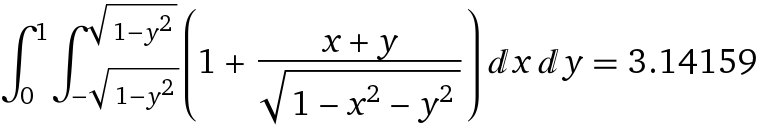## # Wolfram Alpha

Wolfram Alpha (opens new window) 是 Wolfram 公司的在线产品，具有 web 版（免费版功能较少）、移动端 app。

Wolfram Alpha 里面一般只写一个语句，基本不能进行编程。

### # 二重积分

``````integrate((x+y)/sqrt(1-x^2-y^2)+1,[y,0,1],[x,-sqrt(1-y^2),sqrt(1-y^2)])
``````## # Wolfram Mathematica

Mathematica 是一个强大的计算软件，能够在 PC 上进行计算，也可以进行编程。